# (5) Let W denote the set of smooth functions f(2) in CⓇ such that f'(x) =...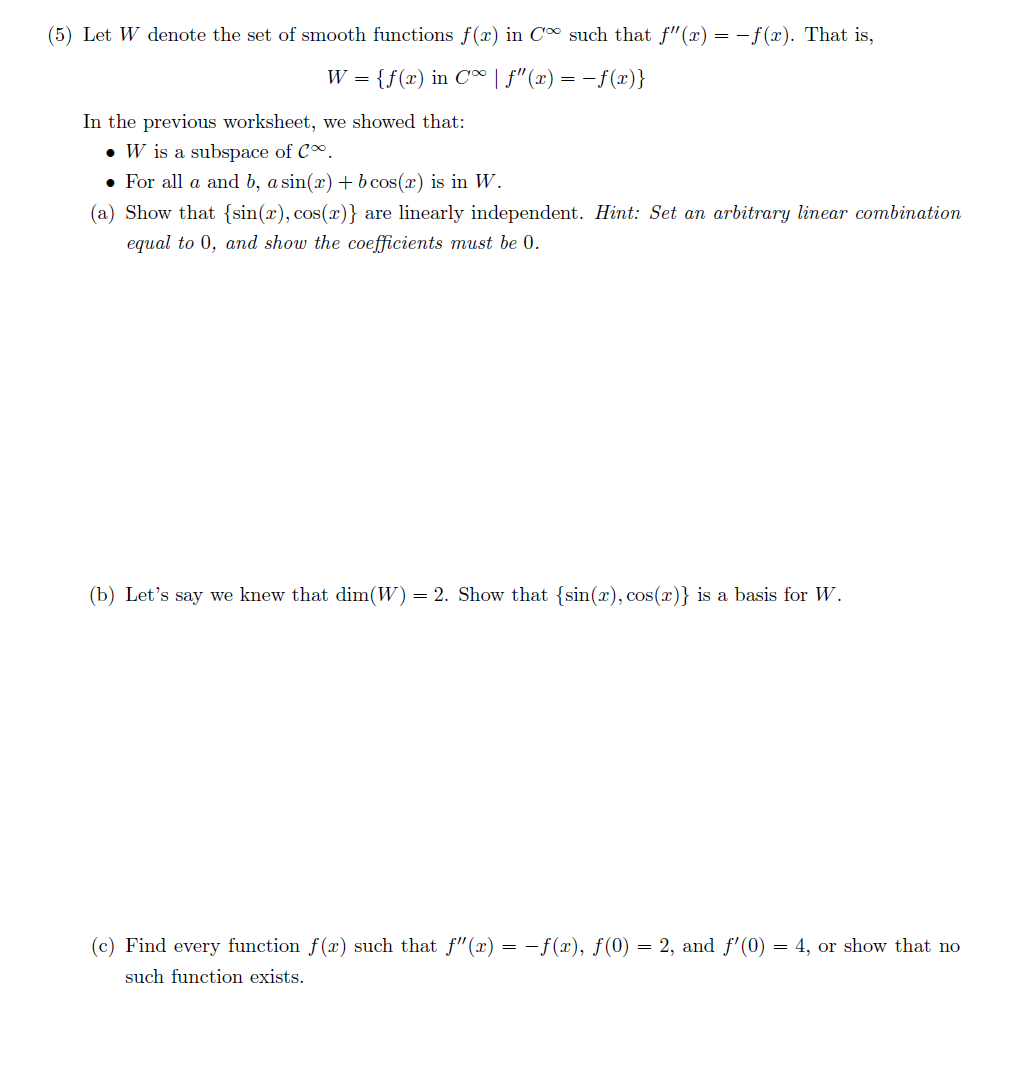(5) Let W denote the set of smooth functions f(2) in CⓇ such that f'(x) = -f(L). That is, W= {f() in C | F"(x) = -f(x)} In the previous worksheet, we showed that: • W is a subspace of Cº. . For all a and b, a sin(2) + b cos(x) is in W. (a) Show that (sin(x), cos(x)} are linearly independent. Hint: Set an arbitrary linear combination equal to 0, and show the coefficients must be 0. (b) Let's say we knew that dim(W) = 2. Show that (sin(x), cos(2)} is a basis for W. (c) Find every function f(x) such that f'(2) = -f(2), f(0) = 2, and f'(0) = 4, or show that no such function exists.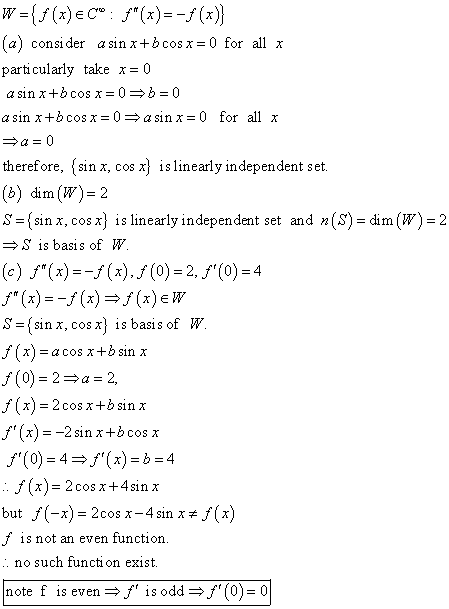##### Add Answer of: (5) Let W denote the set of smooth functions f(2) in CⓇ such that f'(x) =...
Similar Homework Help Questions
• ### Let W denote the set of smooth functions f(x) in CⓇ such that f"(x) = -f(x)....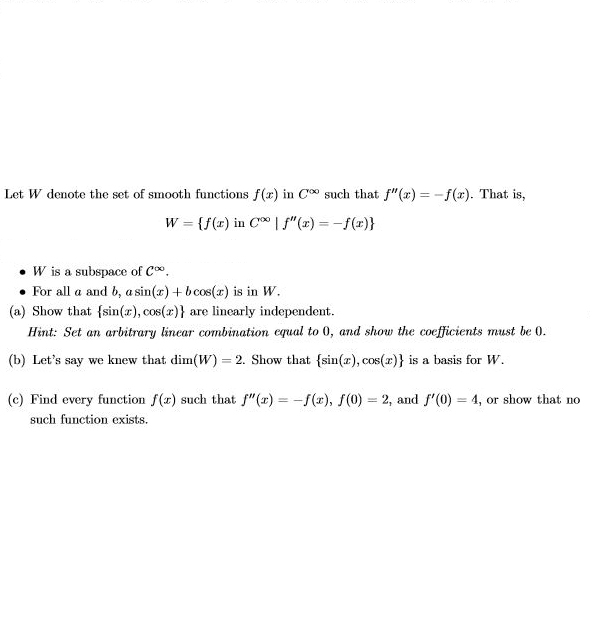Let W denote the set of smooth functions f(x) in CⓇ such that f"(x) = -f(x). That is, W = {f(x) in "S"(t) = -f(x)} . W is a subspace of C . For all a and b, a sin(x) + bcos(x) is in W. (a) Show that (sin(x), cos(x)} are linearly independent. Hint: Set an arbitrary linear combination equal to 0, and show the coefficients must be 0. (b) Let's say we knew that dim(W)=2. Show that (sin(x),cos(x)} is...

• ### Let Coo denote the set of smooth functions, ie, functions f : R → R whose nth derivative exists, ...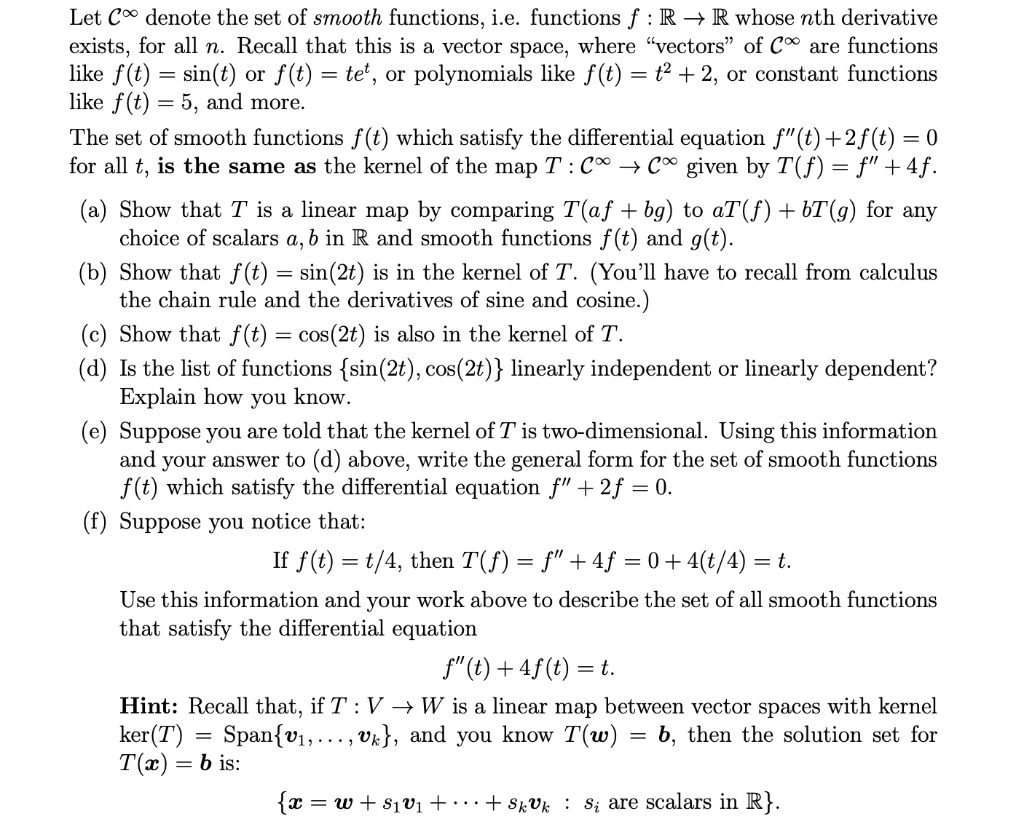Let Coo denote the set of smooth functions, ie, functions f : R → R whose nth derivative exists, for all n. Recall that this is a vector space, where "vectors" of Coo are function:s like f(t) = sin(t) or f(t) = te, or polynomials like f(t)-t2-2, or constant functions like f(t) = 5, and more The set of smooth functions f (t) which satisfy the differential equation f"(t) +2f (t) -0 for all t, is the same as the...

• ### Given the function f(x) = 4x +5 defined on the interval (0, 3, denote by fe...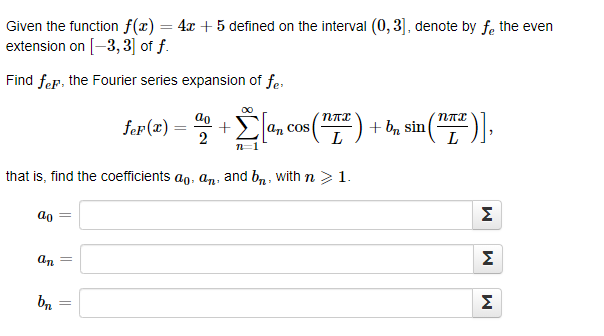Given the function f(x) = 4x +5 defined on the interval (0, 3, denote by fe the even 3,3 of f extension on Find fep, the Fourier series expansion of fe плг пте ao + 2 bsin fer (x) а, COs n-1 that is, find the coefficients ao an, and bn With n> 1 ao ат W |1 l Given the function f(x) = 4x +5 defined on the interval (0, 3, denote by fe the even 3,3 of f...

• ### 2. Let (a, b) nonvanishing. Denote the Frenet frame by {T, N, B} vector a E R3 with R3 be smooth with = 1 and curvature...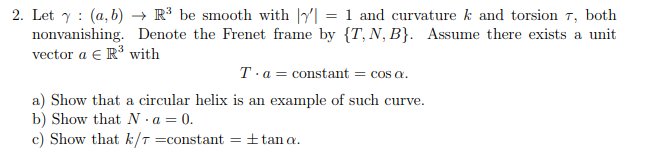2. Let (a, b) nonvanishing. Denote the Frenet frame by {T, N, B} vector a E R3 with R3 be smooth with = 1 and curvature k and torsion r, both Assume there exists a unit Ta constant = COS a. circular helix is an example of such curve a) Show that b) Show that N -a 0. c) Show that k/T =constant ttan a 2. Let (a, b) nonvanishing. Denote the Frenet frame by {T, N, B} vector a...

• ### Problem 2 (Eigenvalues and Eigenvectors). (a) If R2 4 R2 be defined by f(x,y) (y,x), then find al...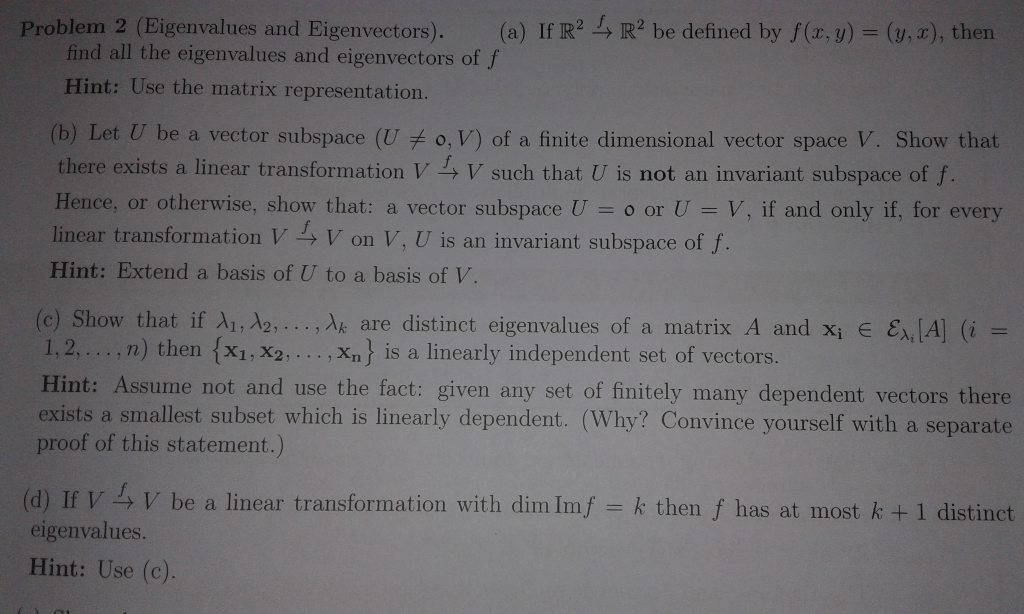Problem 2 (Eigenvalues and Eigenvectors). (a) If R2 4 R2 be defined by f(x,y) (y,x), then find all the eigenvalues and eigenvectors of f Hint: Use the matrix representation. (b) Let U be a vector subspace (U o, V) of a finite dimensional vector space V. Show that there exists a linear transformation V V such that U is not an invariant subspace of f Hence, or otherwise, show that: a vector subspace U-0 or U = V, if and...

• ### Suppose f(x,y) is such that V f is continuous everywhere. Let C be the smooth curve...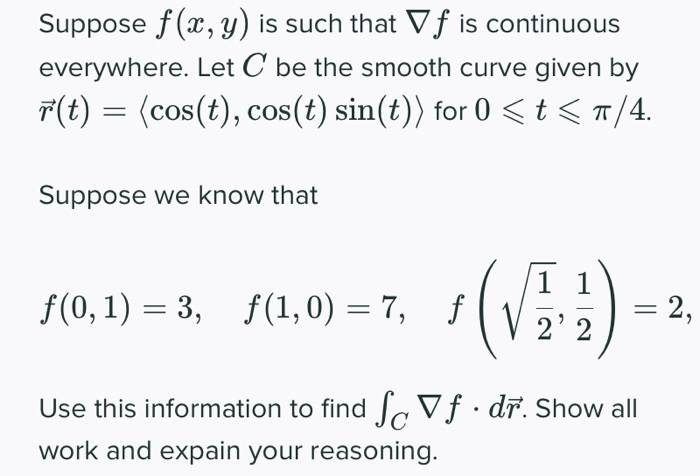Suppose f(x,y) is such that V f is continuous everywhere. Let C be the smooth curve given by F(t) = (cos(t), cos(t) sin(t)) for 0 <t< 7/4. Suppose we know that f(0, 1) = 3, \$(1,0) = 7, f (VE) = 2, 2' 2 Use this information to find Sc Vf. dr. Show all work and expain your reasoning.

• ### We say that an nxn matrix is skew-symmetric if A^T=-A. Let W be the set of...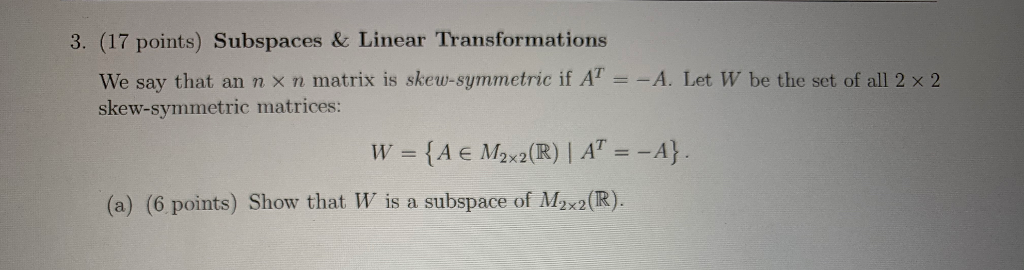We say that an nxn matrix is skew-symmetric if A^T=-A. Let W be the set of all 2x2 skew-symmetric matrices: W = {A in m2x2(R) l A^T=-A}. (a) Show that W is a subspace of M2x2(R) (b) Find a basis for W and determine dim(W). (c) Suppose T: M2x2(R) is a linear transformation given by T(A)=A^T +A. Is T injective? Is T surjective? Why or why not? You do not need to verify that T is linear. 3. (17 points)...

• ### Implicit Function Theorem in Two Variables: Let g: R2 → R be a smooth function. Set {(z, y) E R2 ...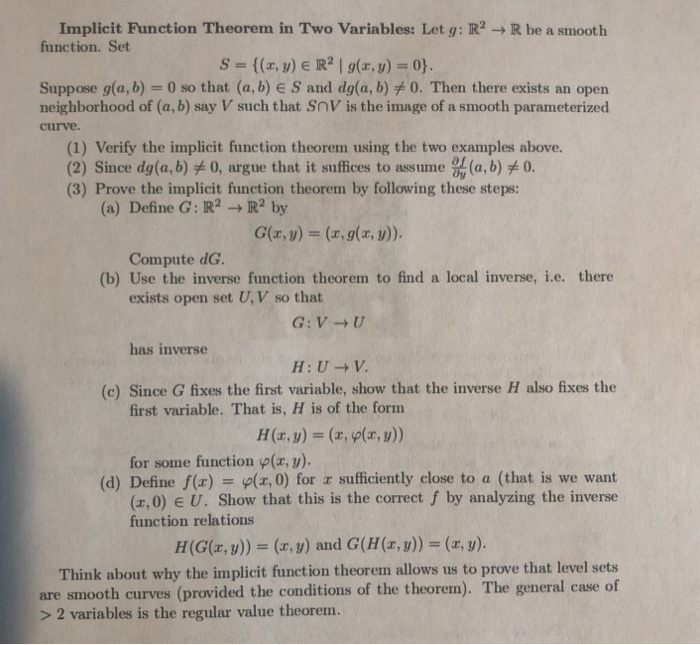Implicit Function Theorem in Two Variables: Let g: R2 → R be a smooth function. Set {(z, y) E R2 | g(z, y) = 0} S Suppose g(a, b)-0 so that (a, b) E S and dg(a, b)メO. Then there exists an open neighborhood of (a, b) say V such that SnV is the image of a smooth parameterized curve. (1) Verify the implicit function theorem using the two examples above. 2) Since dg(a,b) 0, argue that it suffices to...

• ### Consider the function f defined on the interval-5, 5 as follows, { E5,0), те (0,5). 3....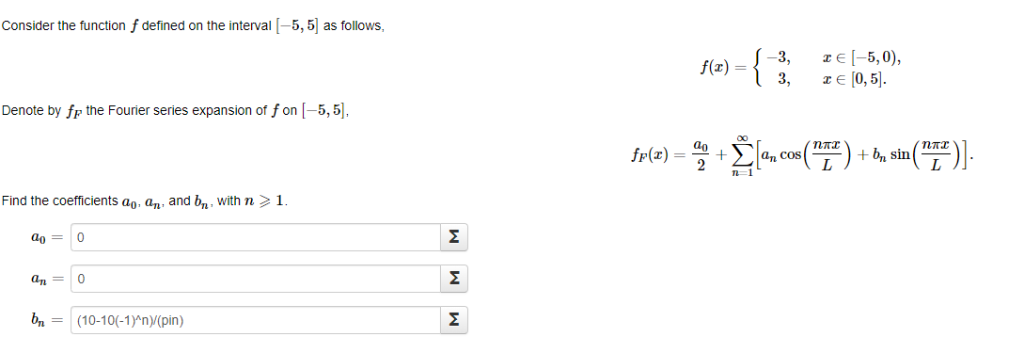Consider the function f defined on the interval-5, 5 as follows, { E5,0), те (0,5). 3. f(x) = 3. Denote by fr the Fourier series expansion of fon [-5,5]. fF(x)= 2 +b sin а, cos Find the coefficients a, an and b with n> 1. 0 an b = (10-10(-1)^n)/(pin) M M M Consider the function f defined on the interval-5, 5 as follows, { E5,0), те (0,5). 3. f(x) = 3. Denote by fr the Fourier series expansion of...

• ### More generally, let f(x) be smooth with f"(xo) formula 0. Show that the truncation error in the with hi = h and ho = h/2 must decrease linearly, and not faster, as h → 0. More generally, let...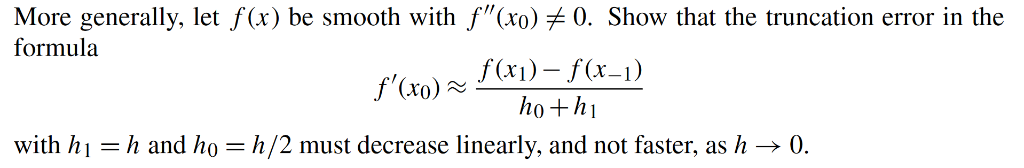More generally, let f(x) be smooth with f"(xo) formula 0. Show that the truncation error in the with hi = h and ho = h/2 must decrease linearly, and not faster, as h → 0. More generally, let f(x) be smooth with f"(xo) formula 0. Show that the truncation error in the with hi = h and ho = h/2 must decrease linearly, and not faster, as h → 0.

Free Homework App